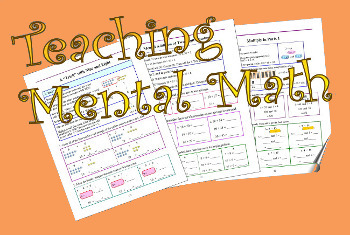Maria's Math News, January 2017

WELCOME to a new year!

In this edition:
1. Math Mammoth news
2. 2017 Mathematics Game (grades 3-12)
3. Practical tips for mental math (grades 1-12)
4. Meaning of factors in multiplication: four groups of 2, or 4 taken two times? (grades 3-6)

## 1. Math Mammoth news

SALE at MathMammoth.com site! Get all the downloads and CDs sold directly on my site at 25% off!

Use coupon code JAN25 at the checkout (via Comecero's system).

This promotion runs through January 25, 2017.

For those interested... the lesson plan for grade 7 (goes to the Homeschool Planet planner) is now available!

In case you're not familiar with these lesson plans, they work in the planner software called "Homeschool Planet", sold by the Homeschool Buyers Co-op.

REMINDER: Math Mammoth printed books and CDs are on sale for 2 more days at Rainbow Resource Center.## 2. 2017 Mathematics Game is here!

It's always FUN!

If you don't know what it is, it's a number "game" or challenge where you build each of the numbers from 1 to 100 using the digits 2, 0, 1, and 7 — and you HAVE to use all those digits!

For example:
10 = 2 + 0 + 1 + 7

8 = 2 × 0 + 1 + 7

7 = 2 × 0 + 1 × 7

...and so on. I'm sure you get the gist!

Read the complete rules for this game here.

## 3. Practical tips for mental mathYou've surely heard of MENTAL MATH, but why is it important for children to learn it?

Because mental math relates to number sense — the ability to manipulate numbers in one's head in various ways in order to do calculations. And number sense has been proven to predict a student's success in algebra.

People with number sense use numbers flexibly. They are able to take them apart and put them together in various ways in order to do calculations. It is quite similar to being able to "PLAY" with words in order to make interesting sentences, or being able to play with chords and melodies in order to make songs.

Read this new article of mine for some mental math strategies that EVERYONE can benefit from!

## 4. Meaning of factors in multiplication: four groups of 2, or 4 taken two times?

Someone asked me about the interpretation or meaning of the two numbers in multiplication, such as 4 × 2. Do you view it as
four groups of 2, OR as four, taken two times?

Here's their question:
"...I noticed that your explanation about how to interpret a math phrase, such as 4 × 2, is different from how I have understood it and taught it to my older daughter who was using RightStart Math at this level.

Let me explain: I see that you are using the first factor to denote number of groups and the second factor tells how many are in each group (4 × 2 would be interpreted 4 groups of 2). On the other hand, I have taught and thought of it reversed, with the first factor telling how many are in each group and the second factor telling you how many groups (4 × 2 would be 4 taken 2 times). I know that the difference in explanations does not ultimately change the product, but I am wondering what you thoughts are. When I conceptually picture what I'm doing I see it as 4 taken 2 times and I feel a little dyslexic trying to see it the other way."

Here's my response:

I'll try to briefly explain the main reason for teaching multiplication that way.

It is because then it becomes easier to tie the concept of multiplication in with OTHER CONCEPTS coming up in the next grades.

Later, students will study multiplication with fractions and decimals, and ultimately with percentages. When we transition to fractions, we see calculations such as 1/2 × 7. I teach students to think of it as "half of seven", in essence "translating" the multiplication sign into "of". It matches the notion of 5 × 7 meaning five groups of seven.

Then when we get to decimals and, most importantly, percentages, we will have 0.3 × 50 which is the same as 30% × 50, or 30% of 50. Notice the word "of" once again. In other words, 30% of 50 is equal to 0.3 × 50. This last idea is of paramount importance for students in algebra, so that they can write algebraic equations for problems involving percentages... for example, to solve for the initial or final price when a discount percentage is known.

One quick example of the above... A gadget was discounted by 45% and now its price is \$67.38. What was the original price? We can write the equation 0.55p = 67.38 and solve it in a snap. The expression 0.55p means 55% OF the original price.

So that is why, in 3rd grade, I teach children to view 5 × 7 as five groups OF seven instead of five, taken seven times. I'm trying to make it easier for them to understand (1) fraction multiplication, (2) percentages, and (3) algebra.

Hope this helps!

Thanks for reading!Feel free to forward this issue to a friend/colleague! Subscribe here.

Till next time,
Maria Miller

--